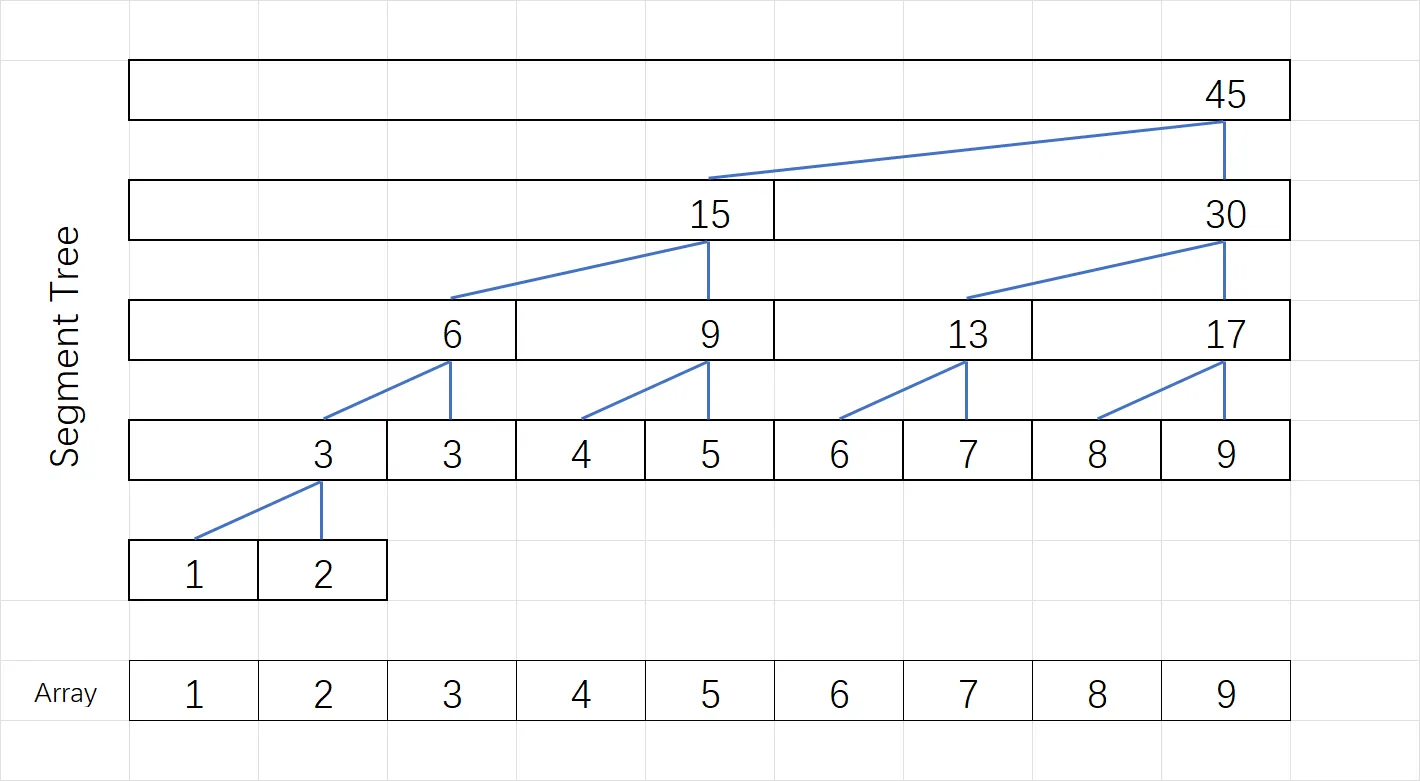• 查找区间 $[l,r]$ 内的大小范围在 $[a,b]$ 的数的个数（类似条件均可查找）
• 查找区间 $[l,r]$ 内第 $k$ 大的数

# 思路# 应用

#### 查找区间 $[l,r]$ 内的 $\leq x$ 的数的个数

• 如果线段树内的区间 $[s,t]$ 完全被 $[l,r]$ 包含，在该节点的有序数列中二分找到 $\leq x$ 的数的个数，加入答案。
• 否则，则考察它的左右子区间，令分界点 $m=\lfloor\frac{s+t}{2}\rfloor$：

• 如果 $[s,t]$ 的左子区间 $[s,m]$ 与 $[l,r]$ 有交集（$l\leq m$）

• 递归考察 $[s,m]$ 区间
• 如果 $[s,t]$ 的右子区间 $[m+1,t]$ 与 $[l,r]$ 有交集（$m+1\leq r$）

• 递归考察 $[m+1,t]$ 区间
• 如果上面的情况都不满足，则说明 $[s,t]$ 区间与我们要求的 $[l,r]$ 区间完全不相交，直接跳过。

#### 查找区间 $[l,r]$ 内第 $k$ 大的数

• 如果 $pl<pr$ 则执行：

• 中点 $mid=(pl+pr)/2$
• 如果查询 $[l,r]$ 中 $\leq res_{mid}$ 的数的个数 $<k$

• 令 $pl = mid + 1$
• 如果查询 $[l,r]$ 中 $\leq res_{mid}$ 的数的个数 $\geq k$

• 令 $pr=mid$
• 最终，第 $k$ 大的数便是 $res_l$.

# 代码

• 建树：$O(n\log n)$
• 查范围数量：$O(\log^2 n)$
• 查第 $k$ 小：$O(\log^3 n)$
struct MergeSortTree
{
/* ### array index must start from ONE ### */
int n;
vector<vector<int>> tree;

// arr: ori arr, [s, t]: cur seg, x: cur node
void build(const vector<int> &arr, int s, int t, int x)
{
if (s == t)
{
tree[x] = {arr[s]};
return;
}
int m = (s + t) / 2;
build(arr, s, m, 2 * x);
build(arr, m + 1, t, 2 * x + 1);
merge(tree[2 * x].begin(), tree[2 * x].end(),
tree[2 * x + 1].begin(), tree[2 * x + 1].end(),
back_inserter(tree[x]));
}

MergeSortTree(const vector<int> &arr) : n(arr.size())
{
int sz = 1 << (__lg(n) + bool(__builtin_popcount(n) - 1)); // sz = \lceil \log_{2}{n} \rceil
tree.resize(2 * sz);
build(arr, 1, n, 1);
}

// [l, r]: query array interval, [mn, mx]: query value interval, [s, t]: cur seg, x: cur node
int count(int l, int r, int mn, int mx, int s, int t, int x)
{
if (l <= s && t <= r)
return upper_bound(tree[x].begin(), tree[x].end(), mx) - lower_bound(tree[x].begin(), tree[x].end(), mn);
int m = (s + t) / 2, ans = 0;
if (l <= m)
ans += count(l, r, mn, mx, s, m, x * 2);
if (r > m)
ans += count(l, r, mn, mx, m + 1, t, x * 2 + 1);
return ans;
}

// query number of elements in the [l, r] interval that fall within the range [mn, mx]
int count(int l, int r, int mn, int mx)
{
return count(l, r, mn, mx, 1, n, 1);
}

// find the kth smallest number in the [l, r] interval
int count(int l, int r, int k)
{
int pl = 1, pr = n;
while (pl < pr)
{
int mid = (pl + pr) / 2;
if (count(l, r, INT32_MIN, tree[mid]) < k)
pl = mid + 1;
else
pr = mid;
}
return tree[pl];
}
};

# 模板题

Luogu P3834: https://www.luogu.com.cn/problem/P3834

(该题应当用复杂度更优的可持久化线段树来完成，用本文的归并树应当只能 AC 五个点)